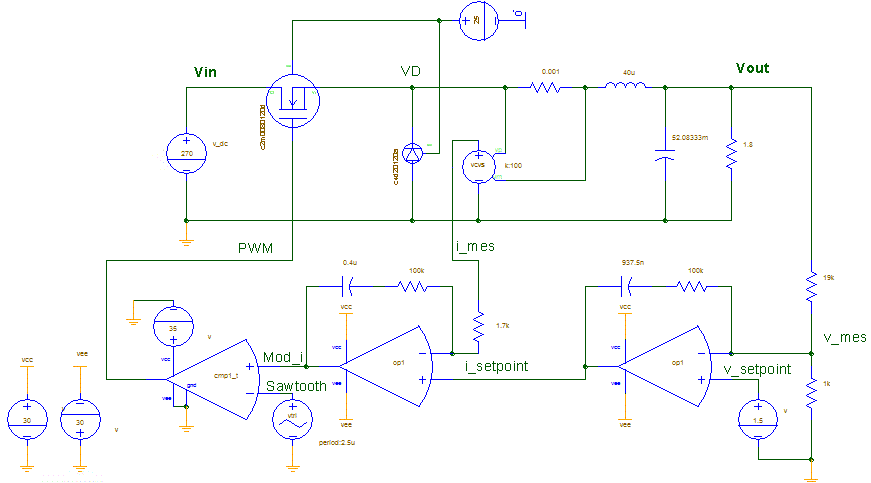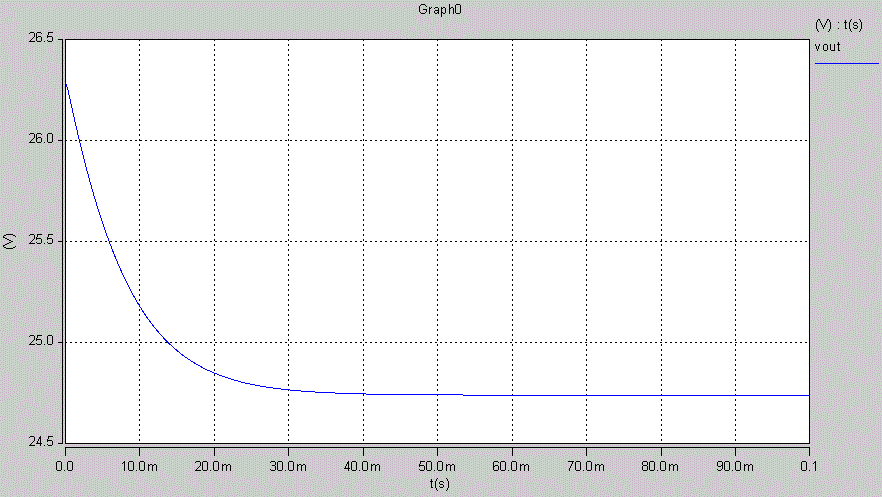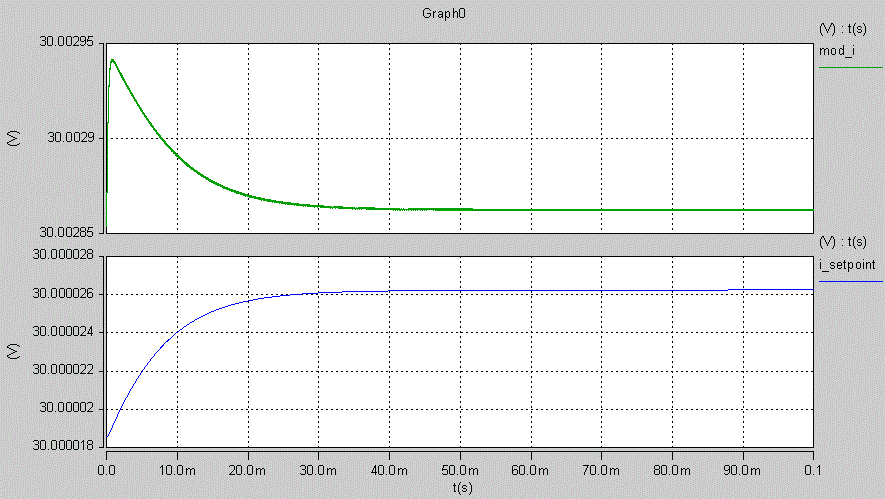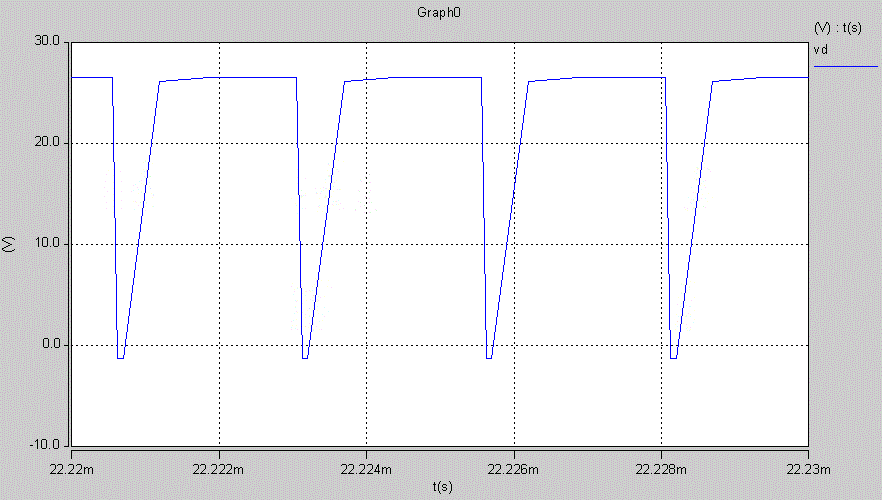Simulation problems of a classical PI controllers using operational amplifiers for buck converters

edited April 2018
Hello,
I would like to simulate a buck converter in closed-loop with SABER. Here is the schematic:Classically, there are two loops with PI controllers: external voltage loop, and internal current loop. The output of the current loop's PI controller is compared to a sawtooth signal in order to generate PWM signals for gate drive. Vin = 270V
Vout_setpoint = 30V
fsw = 400k
time step = 0.5u       However, I obtained the following strange results:
Output voltage:The two op amp are both saturated. (Vcc and Vee are increased to ±30V, which is very big, but if not Vout is very small).The MOSFET's and diode's current are strange, the PWM signals as well:Finally, the diode cathode voltage is not as expected. Its maximum is normally Vin = 270V, but is limited here to 28V:PI parameters are carefully calculated with these two transfert functions:Do you have some ideas to troubleshoot my problem?